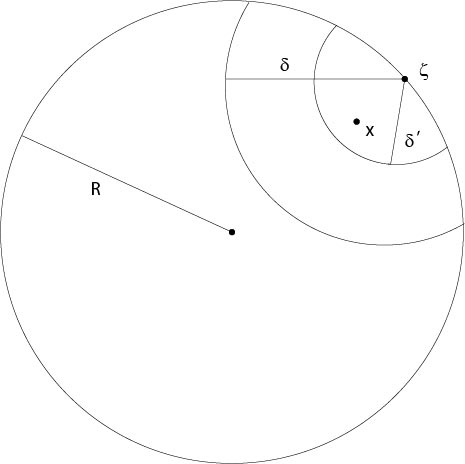$$\newcommand{\id}{\mathrm{id}}$$ $$\newcommand{\Span}{\mathrm{span}}$$ $$\newcommand{\kernel}{\mathrm{null}\,}$$ $$\newcommand{\range}{\mathrm{range}\,}$$ $$\newcommand{\RealPart}{\mathrm{Re}}$$ $$\newcommand{\ImaginaryPart}{\mathrm{Im}}$$ $$\newcommand{\Argument}{\mathrm{Arg}}$$ $$\newcommand{\norm}{\| #1 \|}$$ $$\newcommand{\inner}{\langle #1, #2 \rangle}$$ $$\newcommand{\Span}{\mathrm{span}}$$

# 7.4.1: Green's Function for a Ball

$$\newcommand{\vecs}{\overset { \rightharpoonup} {\mathbf{#1}} }$$ $$\newcommand{\vecd}{\overset{-\!-\!\rightharpoonup}{\vphantom{a}\smash {#1}}}$$$$\newcommand{\id}{\mathrm{id}}$$ $$\newcommand{\Span}{\mathrm{span}}$$ $$\newcommand{\kernel}{\mathrm{null}\,}$$ $$\newcommand{\range}{\mathrm{range}\,}$$ $$\newcommand{\RealPart}{\mathrm{Re}}$$ $$\newcommand{\ImaginaryPart}{\mathrm{Im}}$$ $$\newcommand{\Argument}{\mathrm{Arg}}$$ $$\newcommand{\norm}{\| #1 \|}$$ $$\newcommand{\inner}{\langle #1, #2 \rangle}$$ $$\newcommand{\Span}{\mathrm{span}}$$ $$\newcommand{\id}{\mathrm{id}}$$ $$\newcommand{\Span}{\mathrm{span}}$$ $$\newcommand{\kernel}{\mathrm{null}\,}$$ $$\newcommand{\range}{\mathrm{range}\,}$$ $$\newcommand{\RealPart}{\mathrm{Re}}$$ $$\newcommand{\ImaginaryPart}{\mathrm{Im}}$$ $$\newcommand{\Argument}{\mathrm{Arg}}$$ $$\newcommand{\norm}{\| #1 \|}$$ $$\newcommand{\inner}{\langle #1, #2 \rangle}$$ $$\newcommand{\Span}{\mathrm{span}}$$

If $$\Omega=B_R(0)$$ is a ball, then Green's function is explicitly known.

Let $$\Omega=B_R(0)$$ be a ball in $$\mathbb{R}^n$$ with radius $$R$$ and the center at the origin. Let $$x,\ y\in B_R(0)$$ and let $$y'$$ the reflected point of $$y$$ on the sphere $$\partial B_R(0)$$, that is, in particular $$|y||y'|=R^2$$, see Figure 7.4.1.1 for notations.Figure 7.4.1.1: Reflection on $$\partial B_R(0)$$

Set

$$G(x,y)=s(r)-s\left(\frac{\rho}{R}r_1\right),$$

where $$s$$ is the singularity function of Section 7.1, $$r=|x-y|$$ and

$$\rho^2=\sum_{i=1}^ny_i^2,\ \ \ r_1=\sum_{i=1}^n\left(x_i-\frac{R^2}{\rho^2}y_i\right)^2.$$

This function $$G(x,y)$$ satisfies (i)-(iii) of the definition of a Green function. We claim that

$$u(x)=-\int_{\partial B_R(0)} \frac{\partial}{\partial n_y}G(x,y)\Phi\ dS_y$$

is a solution of the Dirichlet problem (7.3.1.1), (7.3.1.2). This formula is also true for a large class of domains $$\Omega\subset\mathbb{R}^n$$, see .

Lemma.

$$-\frac{\partial}{\partial n_y}G(x,y)\bigg|_{|y|=R}=\frac{1}{R\omega_n}\frac{R^2-|x|^2}{|y-x|^n}.$$

Proof. Exercise.

Set
\begin{equation}
\label{ell13}\tag{7.4.1.1}
H(x,y)=\frac{1}{R\omega_n}\frac{R^2-|x|^2}{|y-x|^n},
\end{equation}
which is called Poisson's kernel.

Theorem 7.2. Assume $$\Phi\in C(\partial\Omega)$$. Then

$$u(x)=\int_{\partial B_R(0)}\ H(x,y)\Phi(y)\ dS_y$$

is the solution of the first boundary value problem (7.3.1.1), (7.3.1.2) in the class $$C^2(\Omega)\cap C(\overline{\Omega})$$.

Proof. The proof follows from following properties of $$H(x,y)$$:

1. $$H(x,y)\in C^\infty,\ \ |y|= R,\ |x|<R,\ x\not=y$$,
2. $$\triangle_xH(x,y)=0,\ \ |x|<R,\ |y|=R$$,
3. $$\int_{\partial B_R(0)}\ H(x,y)\ dS_y=1,\ \ |x|<R$$,
4. $$H(x,y)>0,\ \ |y|=R,\ |x|<R$$,
5. Fix $$\zeta\in\partial B_R(0)$$ and $$\delta>0$$, then $$\lim_{x\to\zeta,|x|<R}H(x,y)=0$$ uniformly in $$y\in\partial B_R(0),\ |y-\zeta|>\delta$$.

(i), (iv) and (v) follow from the definition (\ref{ell13}) of $$H$$ and (ii) from (\ref{ell13}) or from

$$H=-\frac{\partial G(x,y)}{\partial n_y}\bigg|_{y\in\partial B_R(0)},$$

$$G$$ harmonic and $$G(x,y)=G(y,x)$$.

Property (iii) is a consequence of formula

$$u(x)=\int_{\partial B_R(0)}\ H(x,y)u(y)\ dS_y,$$

for each harmonic function $$u$$, see calculations to the representation formula above. We obtain (ii) if we set $$u\equiv1$$.

It remains to show that $$u$$, given by Poisson's formula, is in $$C(\overline{B_R(0)})$$ and that $$u$$ achieves the prescribed boundary values. Fix $$\zeta\in \partial B_R(0)$$ and let $$x\in B_R(0)$$. Then

\begin{eqnarray*}
u(x)-\Phi(\zeta)&=&\int_{\partial B_R(0)}\ H(x,y)\left(\Phi(y)-\Phi(\zeta)\right)\ dS_y\\
&=& I_1+I_2,
\end{eqnarray*}
where
\begin{eqnarray*}
I_1&=&\int_{\partial B_R(0),\ |y-\zeta|<\delta}\ H(x,y)\left(\Phi(y)-\Phi(\zeta)\right)\ dS_y\\
I_2&=&\int_{\partial B_R(0),\ |y-\zeta|\ge\delta}\ H(x,y)\left(\Phi(y)-\Phi(\zeta)\right)\ dS_y.\\
\end{eqnarray*}
For given (small) $$\epsilon>0$$ there is a $$\delta=\delta(\epsilon)>0$$ such that

$$|\Phi(y)-\Phi(\zeta)|<\epsilon$$

for all $$y\in\partial B_R(0)$$ with $$|y-\zeta|<\delta$$. It follows $$|I_1|\le\epsilon$$ because of (iii) and (iv). Set $$M=\max_{\partial B_R(0)}|\phi|$$. From (v) we conclude that there is a $$\delta'>0$$ such that

$$H(x,y)<\frac{\epsilon}{2M\omega_nR^{n-1}}$$

if $$x$$ and $$y$$ satisfy $$|x-\zeta|<\delta',\ |y-\zeta|>\delta$$, see Figure 7.4.1.2 for notations.Figure 7.4.1.2: Proof of Theorem 7.2

Thus $$|I_2|<\epsilon$$ and the inequality

$$|u(x)-\Phi(\zeta)|<2\epsilon$$

for $$x\in B_R(0)$$ such that $$|x-\zeta|<\delta'$$ is shown.

$$\Box$$

Remark. Define $$\delta\in[0,\pi]$$ through $$\cos\delta=x\cdot y/(|x||y|)$$, then we write Poisson's formula of Theorem 7.2 as

$$u(x)=\frac{R^2-|x|^2}{\omega_nR}\int_{\partial B_R(0)}\Phi(y)\frac{1}{\left(|x|^2+R^2-2|x|R\cos\delta\right)^{n/2}}\ dS_y.$$

In the case $$n=2$$ we can expand this integral in a power series with respect to $$\rho:=|x|/R$$ if $$|x|<R$$, since

\begin{eqnarray*}
\frac{R^2-|x|^2}{|x|+R^2-2|x|R\cos\delta}&=&\frac{1-\rho^2}{\rho^2-2\rho\cos\delta+1}\\
&=&1+2\sum_{n=1}^\infty \rho^n\cos(n\delta),
\end{eqnarray*}

see , pp. 18 for an easy proof of this formula, or , Vol. II, p. 246.

## Contributors

• Integrated by Justin Marshall.